American Journal of Optics and Photonics
Volume 3, Issue 4, August 2015, Pages: 48-53

The Ellipsometrical Analysis of External Reflection of Light on Superficial Films on Solid Substrates

Simion Jitian

Politehnica University Timisoara, Faculty of Engineering, Hunedoara, Romania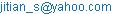(S. Jitian)

Abstract: The ellipsometrical analysis of the external specular reflection of light on nonabsorbing superficial films allows us to know factors which influence the ellipsometric measurement of the analyzed system. For optical nonabsorbing superficial films the curves D=f(df) and Y=f(df) are periodical, while the curves D=f(Y) are closed. The paper presents observations on the dependence of the ellipsometric parameters D and Y on df. The analysis of the periodicity of these curves allows us to correctly determine the film thickness for thicknesses greater than dmin. The value of dmin depends on the refractive index nf of the surface film, the incidence angle φ0 and the wavelength λ of the incident radiation. The dependence of dmin on nf, φ0 and λ is analyzed. From the curve shape we can draw conclusions with respect to the domain of small errors, allowing us to correctly determine the thickness and refraction index of superficial films.

Keywords: Ellipsometry, Superficial Film, External Specular Reflection

1. Introduction

In the case of reflection of monochromatic light radiation on the surface of a homogeneous and isotropic, optically non-absorbent surface film deposited on a solid support reflector, there is a change of the polarization state of light. Figure 1 is the schematic model of the external reflection of monochromatic radiation on an optically absorbent surface film.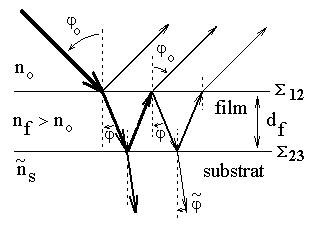Figure 1. The external reflection of a monochromatic plane polarized radiation on the substrate-film surface.

The modification of the polarization state of the reflected radiation can be ellipsometrically measured . D and Y are the parameters that can be measured. The D parameter expresses the phase angle between the light components, parallel to the plane of incidence and perpendicular to the plane of incidence, after reflection. Here, tanY is the relative attenuation of two components, after reflection.

The complex parameter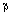can be calculated depending on the parameters D and Y by the relation: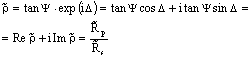(1)

by taking into account the reflections and therefore the attenuation and phase shift on all interfaces.

The complex parameters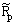and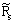are expressed in terms of complex Fresnel reflection coefficients corresponding to reflections on the two interfaces S12 and S23: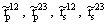:and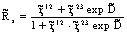(2)

where: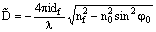(3)

The expression: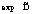is a regular function that depends on the thickness and refractive index of the film surface. Also, that expression depends on incidence angle and wavelength of the incidence radiation.

2. The Dependence of the Ellipsometrical Parameters D and Y on Superficial Film Thickness

In the case of external reflection, nf>n0. The complex parameters: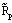,and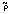should be repeated after a particular value of the film thickness df, for which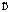is a multiple of 2pi.

The ellipsometrical readings D and Y, calculated from relations: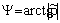(4)

are also periodical functions of the superficial film thickness, having the same period. For this reason, the curves D = f(Y), shown in Figure 2, are closed loops .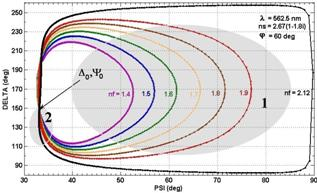Figure 2. The curves D = f(Y) for superficial films with nf=1.2÷2.5, deposited on a metallic substrate.  ns=2.67(1-1.18i); j0=600; l=562.5 nm. D0 and Y0 are the ellipsometric measured parameters for the substrate free of film.

Figure 3 shows the variation of the ellipsometrical readings D and Y according to the superficial film thickness.

The minimum of film thickness for which the exponential function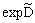is repeated is dmin.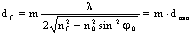; m= 1, 2, 3,…    (5)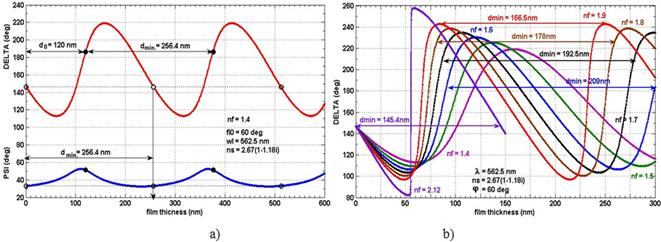Figure 3. The curves D = f(df) and Y = f(df) for superficial films, deposited on a metallic substrate. ns=2.67(1-1.18i); j0=600; l=562.5 nm.

The film thickness df is real because nf>n0. The minimum film thickness for which values of D and Y is repeated is dmin.

You can determine the exact thickness of an optical non-absorbent film, by ellipsometrical measurements, only if it is thinner than dmin. For thicker films, the thickness shall be expressed by the relationship: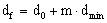(6)

where d0 is the thickness, determined by ellipsometrical measurement, and m is an integer. For example, for D=186.370 and Y=51.3170, the film thickness with nf=1.4 can be 120nm or df = (120 + m·256.4) nm; m = 1, 2, 3,...  as shown in Figure 3a.

For films with thicknesses greater than dmin, further measurements are needed to determine the thickness, due to the periodicity of the functions D=f(df)  and Y=f(df). Usually, interferometer measurements are used to determine the order of magnitude of df, with a precision of 0.1 mm [4,5,6]. Typically, the thickness dmin value is on the order of 0.3¸0.5 mm. It is then necessary to determine the exact thickness, by an ellipsometrical measurement, with an accuracy of 0.1 nm.

The value of dmin depends on the refractive index nf of the surface film as well as on the measuring conditions: the incidence angle φ0 and the wavelength λ of the incident radiation, according to the relation (5).

2.1. The Dependence of dmin on the Refractive Index nf

Table 1. The values of minimum thickness dmin of the surface film (expressed in nm) for which the ellipsometrical parameters  D and Y is repeated.

 λ φ0 nf nm deg 1.4 1.6 1.8 2.0 2.12 2.2 562.5 50 240.0 200.2 172.7 152.2 142.3 136.4 60 255.7 209.1 178.2 156.0 145.3 139.1 70 271.0 217.2 183.2 159.3 148.0 141.4 75 277.5 220.5 185.2 160.6 149.0 142.3 80 282.6 223.0 186.7 161.6 149.8 143.0

When increasing the refractive index nf of the superficial film the value of dmin decreases, as shown in Figure 3b and in Table 1.

The curves Y = f (df) reach a peak (Y = 900) for a particular value of the refractive index of the film and for a particular value of its thickness, as shown in Figure 4.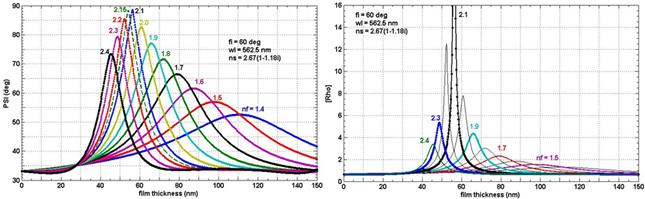Figure 4. The curves Y = f(df) and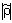= f(df) for superficial films, deposited on a metallic substrate. ns=2.67(1-1.18i); j0=600; l=562.5 nm.

Then,(7)

or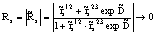(8)

or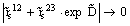(9)

The maximum of the curve Y=f(df) = 900 is obtained for nΨmax = 2.12 and dΨmax=55.4nm.

Figure 5 presents the slopes of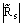and its first derivative depending on the superficial film thickness.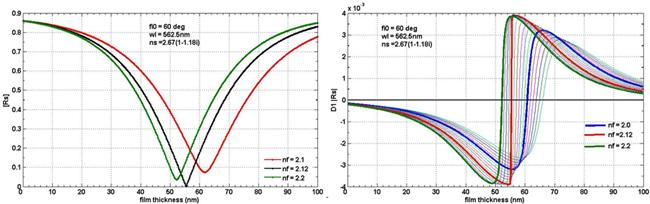Figure 5. The curves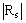= f(df)  and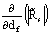= f(df)  for superficial films, on a metallic substrate. ns=2.67(1-1.18i); j0=600; l=562.5 nm.

At the point where Y=900,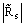=0. The curve= f (df) suddenly changes its concavity and the first derivative of the modulus of complex parameteris indefinite. The ellipsometric parameter D is also indefinite, as shown in Figure 3b.

The curves=f(df), Real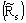=f(df) and Imagare presented in Figure 6.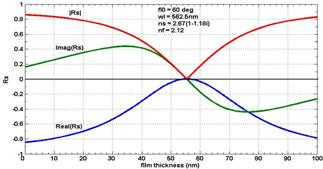Figure 6. The curves=f(df) , Real=f(df) and Imagfor superficial films, deposited on a metallic substrate. nf = 2.12; ns=2.67(1-1.18i); j0=600; l=562.5 nm.

Information regarding the errors in the determination of the thickness and refractive index of a surface film is obtained by deriving the ellipsometric parameters D or Y according to them.

The first derivative of D and Y depending on the superficial film thickness D1D and D1Y are plotted in Figure 7.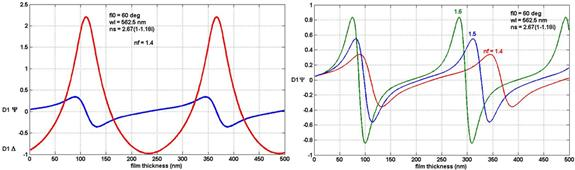Figure 7. The first derivative of D and Y, depending on the superficial film thickness, deposited on a metallic substrate.  ns=2.67(1-1.18i); j0=600; l=562.5 nm.

One can notice that D1Y = 0 when the variation of D is maximum and D1D = 0 when the variation of  Y is maximum. Minimum errors in determining the refractive index nf and the thickness df of superficial film are obtained when both ellipsometric parameters D and Y  shows large variations while the thickness or refractive index of the film is changing (the shaded area 1, in Figure 2a).

For superficial films with thicknesses close to dmin value, or multiple of this, the error in the determination of nf is very high (the shaded area 2 of Figure 2a).

2.2. The Dependence of dmin on the Incidence Angle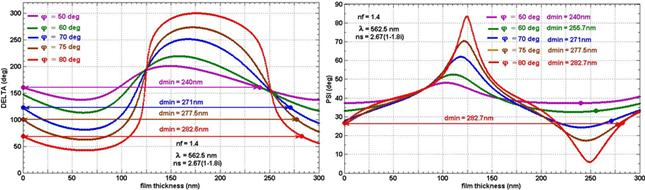Figure 8. The curves D = f(df) and Y = f(df) for superficial films, deposited on a metallic substrate, for different incidence angles. ns=2.67(1-1.18i); l=562.5 nm.

The minimum thickness dmin after which Y and D are repeated, increases when the angle of incidence φ0 of the incident radiation increases, as shown in Figure 8, according to the relation (4).

An analysis of the dependence of the reflection coefficientsandon the incidence angle was performed by R.MA Azzam et al [7,8,9].

2.3. The Dependence of dmin on the Wavelength

Thickness dmin increases when there are increases of wavelength λ, as shown in Figure 9, according to the relation (4).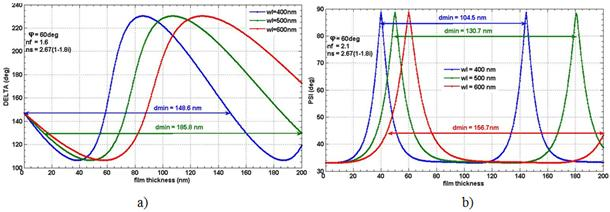Figure 9. The curves D = f(df) and Y = f(df) for superficial films, deposited on a metallic substrate, at different wavelengts. ns=2.67(1-1.18i); j0=600; a)nf=1.6; b) (Ψ=Ψmax=900 for the same nΨmax = 2.12 irrespective of wavelength λ).

The analysis of these curves provides the following observations:

Ÿ The curves D = f (df) and Y = f (df) have the same amplitude for a given value of the refractive index of the surface film.

Ÿ The film thickness dΨmax, for which Ψ=900, depends on the wavelength of the incident radiation. It increases when λ increases. The ratio dΨmax/λ is constant. dΨmax = k·λ.

Ÿ The value of the refractive index nΨmax of the superficial film for which Ψ=Ψmax=900 does not depend on λ.

Ÿ When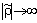or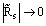the value of Ψmax=900 is obtained. It does not depend on the wavelength λ. The curves=f(df) for superficial films with refractive index nΨmax, at different wavelengths are presented in figure 10.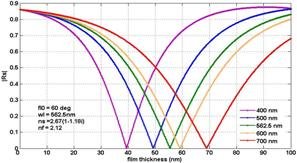Figure 10. The curves=f(df)  for superficial films, on a metallic substrate, at different wavelengths; nΨmax = 2.12; ns=2.67(1-1.18i); j=600.

Ÿ For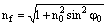;    dmin=l/2                             (10)

Ÿ For non-absorbing superficial films with nf=1.3÷1.6, always dmin.<l.

All computations were performed based on the Fortran program developed by McCrackin, amended as necessary . The graphs were performed with the Matlab software.

3. Conclusions

Ellipsometrical analysis of external specular reflection of light on nonabsorbing superficial films allows us to know the factors that influence the ellipsometric measurement of the analyzed system. The right choice of the angle of incidence φ0 and wavelength λ depending on the refraction index of the superficial film, ellipsometrically measured, allows to be obtained minimal errors in the determination of its thickness and refraction index.

For optical nonabsorbing superficial films the curves D=f(df) and Y=f(df) are periodical, while the curves D=f(Y) are closed. The analysis of the periodicity of these curves allows us to correctly determine the film thickness for thicknesses greater than dmin. Knowing the minimal value of the film thickness from which the values of D and Y are repeated eliminates possible errors in the determination of the superficial film thickness.

The value of dmin depends on the refractive index nf of the surface film, the incidence angle φ0 and the wavelength λ of the incident radiation.

The particular situation under analysis corresponds to a value of the ellipsometric parameter Y=900. Then, or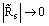. Useful applications of this interesting property are yet to be considered.

From the curve shape we can draw conclusions with respect to the domain of small errors, allowing us to correctly determine the thickness and refraction index of superficial films.

Applications of these results are possible in the measurement and control of film thickness.

The results obtained testify that the materials with the refractive index close to 1.8÷2 can be considered for photonic crystal application area.

References

1. R.M.A. Azzam, N.M. Bashara, Ellipsometry and Polarized Light, North-Holland publ. Comp., Amsterdam - New York - Oxford, 1977.
2. S. Jitian, "The ellipsometrical study of adsorption-desorption of the corrosion inhibitors on metallic surfaces",Romanian Reports in Physics, Vol.65, No.1, pp. 204–212, 2013.
3. S. Jitian, E. Chifu,, "The Ellipsometric Study of Polimer Films on Metals", Studia Univ. Babeş-Bolyai. Chemia, Vol.31, No. 2, pp.69-75, 1986.
4. H. Rosen, J. Shamir, "Interferometric determination of ellipsometric parameters" J. Phys. E: Sci. Instrum., Vol.11, No.9, pp.905-908, September 1978.
5. K.H. Chen, C.C. Hsu, D.C. Su, "A method for measuring the complex refractive index and thickness of a thin metal film", Appl. Phys. B – Lasers and Optics, Vol.77, pp.839–842, December 2003.
6. Juan E. González-Ramírez, Juan Fuentes, Luis C. Hernández, and Luís Hernández," Evaluation of the thickness in nanolayers using the Transfer Matrix method for modeling the spectral reflectivity",Research Letters in Physics, Vol. 2009, Article ID 594175, 2009.
7. R. M.A. Azzam, M. Emdadur Rahman Khan, "Complex reflection coefficients for the parallel and perpendicular polarizations of a film–substrate system", Applied Optics, Vol.22, No.2, pp.253-264, January 1983.
8. R. M.A. Azzam, T.F. Thonn, "Pseudo-Brewster and second-Brewster angles of an absorbing substrate coated by a transparent thin film", Applied Optics, Vol.22, No.24, pp.4155-4166, December 1983.
9. R.M.A. Azzam, "Angle-of-incidence derivatives of the complex parallel and perpendicular reflection coefficients and their ratio for a film-substrate system "Opt. Acta, Vol.30, No.8, pp.1113-1124, August 1983.
10. F.L. McCrackin, A Fortran Program for Analysis of Ellipsometer Measurement, Natl. Bur. Std., Technical Note 479, Washington, 1969.

 Contents 1. 2. 2.1. 2.2. 2.3. 3.
Article ToolsAbstractPDF(694K)